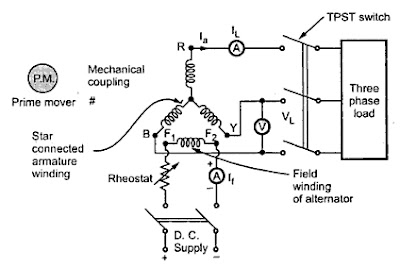Today in this post we are going to learn what is Voltage regulation of synchronous machine and different methods to calculate Voltage regulation of synchronous machine.

## The definition of Voltage regulation of a synchronous machine:

Voltage Regulation of synchronous machine is defined as the difference between the terminal voltage at no load and terminal voltage at full load and excitation, speed must remain same.Voltage Regulation of synchronous machine is generally calculated in percentage of full load terminal voltage.

## Objectives for calculating Voltage regulation of a synchronous machine:

1. Parallel operation of alternators is affected by the voltage regulation. By calculating voltage regulation of synchronous machine we can adjust the parallel operating machines to be in synchronism.
2. Calculating voltage regulation of a synchronous machine determines the type of automatic voltage control equipment required for resisting the voltage changes.
3.When the load is thrown off voltage rise must be known because with the rise in voltage the insulation must be able to withstand this rise.
So the calculation of voltage regulation of synchronous machine has a great importance.

## The general expression for calculating Voltage regulation of synchronous machine:

Now let us derive the general expression for calculating voltage regulation of a synchronous machine
Let E be the terminal voltage of the synchronous machine at no load. Now if the synchronous machine is given full load the terminal voltage will no longer be E because of the losses so let the terminal voltage now be V.
So general expression for Voltage regulation of a synchronous machine is given by
Voltage regulation% = (E – V / V) × 100

## Methods for calculating voltage regulation of synchronous machine:

There are two types of methods for calculating voltage regulation of synchronous machine.
2. Indirect Method.
The indirect method of calculating voltage regulation of synchronous machine can be further classified into 3 types:
1.EMF method or Synchronous impedance method.
2. MMF method or Ampere turn method.
3.Zero power factor method or Potier method.

## Direct load test method for calculating voltage regulation of synchronous machine:

Now let’s see how to calculate voltage regulation of synchronous machine by using direct load test method:

### Circuit diagram for calculating Voltage regulation of synchronous machine by direct load test:### Circuit connections for calculating voltage regulation of synchronous machine by direct load test:

1.Firstly connections are to be made as given in the circuit diagram:
2. Armature which is star connected is connected to the three-phase load with the help of TPST. TPST is a switch and it means triple pole single through.
3. A rheostat is connected in series with the field winding.
4. The field winding is excited by using D.C supply and flux is adjusted by adjusting the rheostat. Flux adjustment is nothing but adjusts the current flow through field winding.

## Procedure for calculating voltage regulation of synchronous machine by direct load test:

1. Adjust the prime mover such that the alternator rotates at synchronous speed Ns.
we know Eph α 𝞍 from emf equation
2. Now DC supply is given to the field winding and the current flow through the field is adjusted so that the flux is adjusted such that the rated voltage is obtained at its terminals which can be seen on the voltmeter connected across the lines.
3. Now load is connected to the alternator with the help of TPST switch.
4.The load is then increased such that the ammeter reads rated current. This is full load condition of the alternator. Now as the load is connected due to armature reaction there is a loss of voltage so let the induced voltage be V.
5.Now again adjust the rheostat of the field winding to get rated voltage at alternator terminals.
6.Now remove the load by opening TPST switch and the excitation, speed should not be changed it should be same as before removing the load.
7. As there is no load there is no armature reaction the induced emf is equal to the terminal voltage which is E.
Now we can calculate voltage regulation of synchronous machine by
Voltage regulation% =( E – V / V) × 100 at a specific power factor.

## Limitations for calculating voltage regulation of synchronous machine by using direct load method:

This method is applicable only for small capacity machines for larger capacity machines it is not economical because that much load cannot be given directly.
In this way, we have calculated the voltage regulation of synchronous machine by direct load test method.
For larger capacity machines voltage regulation can be calculated by the Indirect method.
In the next post, we can see how to calculate voltage regulation of synchronous machine by the Indirect method.